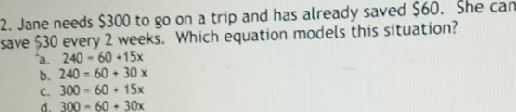### ¿Todavía tienes preguntas de matemáticas?

Pregunte a nuestros tutores expertos
Algebra
Pregunta2. Jone needs $$\ 300$$ to go on a trip and has already saved $$\ 60$$ . She can save $$\ 30$$ every $$2$$ weeks. Which equation models this situation?

a. $$240 - 60 + 15 x$$

b. $$240 = 60 + 30 x$$

c. $$300 - 60 + 15 x$$

d. $$300 - 60 + 30 x$$